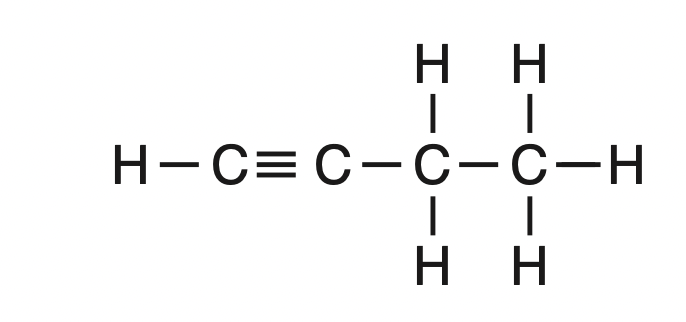top of page
Search

# What is the amount of heat absorbed when the temperature of 75 grams of water increases from 20.°

NY Regents Chemistry Exam June 2019

Questions 41-50

41 What is the amount of heat absorbed when the temperature of 75 grams of water increases from 20.°C to 35°C? (1) 1100 J

(2) 4700 J

(3) 6300 J

(4) 11 000 J

Solution: Table T has equations for heat calculation. If there is a temperature change, the equation is q=mcΔT. c is the specific heat capacity of water and can be found on Table B of the Reference Table. q=75g(4.18 J/g•K)(35°C - 20°C)

42 Which sample of HCl(aq) reacts at the fastest rate with a 1.0-gram sample of iron filings? (1) 10. mL of 1 M HCl(aq) at 10.°C

(2) 10. mL of 1 M HCl(aq) at 25°C

(3) 10. mL of 3 M HCl(aq) at 10.°C

(4) 10. mL of 3 M HCl(aq) at 25°C

Solution: The higher the reactant concentration and the higher the temperature, the faster the rate of reaction will be.

43 Given the equation representing a system at equilibrium: N2O4(g) ⇌ 2NO2(g) Which statement describes the concentration of the two gases in this system?

(1) The concentration of N2O4(g) must be less than the concentration of NO2(g).

(2) The concentration of N2O4(g) must be greater than the concentration of NO2(g).

(3) The concentration of N2O4(g) and the concentration of NO2(g) must be equal.

(4) The concentration of N2O4(g) and the concentration of NO2(g) must be constant.

Solution: At equilibrium the concentration of the reactants and products must be constant.

44 Given the equation representing a system at equilibrium: PCl5(g) + energy ⇌ PCl3(g) + Cl2(g) Which change will cause the equilibrium to shift to the right? (1) adding a catalyst (2) adding more PCl3(g) (3) increasing the pressure (4) increasing the temperature

Solution: Adding a catalyst does shift the equibrium. It just increases the rate of the reaction. Adding more PCl3 will cause shift to the left to get rid of PCl3. Increasing pressure means there is too much gas and we shift to the side with the least moles of gas, which is left. Increasing temperature will shift the equilibrium to the right since energy is on the left and we will want to go to the opposite side to lower it.

45 Given the formula representing a molecule:

A chemical name for this compound is(1) pentanone

(2) 1-pentanol (3) 1-pentanamine

(4) pentanamide

Solution: We can look at Table R on the Reference Table. The functional group here is NH2, which is an amine. Example of the name is given on the right.46 Given the formula of a compound:This compound is classified as an (1) aldehyde

(2) alkene

(3) alkyne

(4) alcohol

Solution: Table Q on the Reference Table shows hydrocarbons. The hydrocarbon that has a triple bond in an alkyne.47 Which equation represents fermentation?

(1) C2H4 + H2O → CH3CH2OH (2) C2H4 + HCl → CH3CH2Cl (3) C6H12O6 → 2CH3CH2OH + 2CO2

(4) 2CH3CHO → C3H5CHO + H2O

Solution: Fermentation reaction produces an alcohol and carbon dioxide.

48 Given the equation representing a reaction:

3CuCl2(aq) + 2Al(s) → 3Cu(s) + 2AlCl3(aq) The oxidation number of copper changes from

(1) +1 to 0

(2) +2 to 0

(3) +2 to +1

(4) +6 to +3

Solution: Let's calculate the oxidation number of copper in CuCl2. Cl has an oxidation number if -1 according to the Periodic Table. The molecule is neutral overall. We can make an equation where x is the oxidation number of copper.

X + 2(-1) = 0

X = +2

An element on its own has an oxidation number of 0. Therefore Cu(s) is 0.

49 Given the equation representing a reversible reaction:

CH3COOH(aq) + H2O(l) ⇌ CH3COO-(aq) + H3O+(aq)

According to one acid-base theory, the two H+ donors in the equation are (1) CH3COOH and H2O

(2) CH3COOH and H3O+

(3) CH3COO- and H2O

(4) CH3COO- and H3O+

Solution: An H+ donor will lose a hydrogen. We can see CH3COOH loses a hydrogen to become CH3COO- and H3O+ loses an H to become H2O.

50 Which nuclear equation represents a spontaneous decay?Solution: In a spontaneous decay equation we will see only one reactant on the left side of the equation.